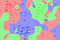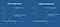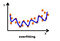# Fundamentals Of Machine Learning Part 5- KNN(K Nearest Neighbors)

In this article we are going to talk about a very famous machine learning algorithm that is used for classification as well as for regression.

‘Birds of a feather flock together’

KNN algorithm works on the assumption that data having similar inputs will have similar outputs. The KNN algorithm assumes that similar things exist in close proximity. In other words, similar things are closer to each other.Similar data point stay close to each other.

Now the question is how to measure similarities between the points?

There are many techniques to find that and you might be familiar with some of them. …

# Fundamentals of Machine Learning part 4- Logistic Regression and PLA

Let’s dive in into the functioning of Logistic Regression and PLA.

Prerequisites :-

Linear Regression and Gradient Descent.

# LOGISTIC REGRESSION :-Logistic Regression uses sigmoid function .

We have talked about linear regression which is useful for regression task. Logistic Regression is used for classification task , where the dependent variable only contains discrete values . Logistic Regression is a “misnomer” . The reason it is called regression because it uses the underlying properties of Linear Regression. Logistic Regression is a child of the root model know as “Generalized Linear Model”.

Logistic Regression tries to find the best “decision boundary” that separates the different classes. …

# Fundamentals Of Machine Learning Part 3 — Regularization In Regression

In this article we are going to implement regularization techniques in linear models/regression.

## Prerequisites :-

• Linear Regression
• Cost Function
• Gradient Descent

If you are not familiar with these terms then consider going through this article:-

Fundamentals Of Machine Learning part 2- Linear Regression | by AKSHAY KUMAR RAY | Analytics Vidhya | Mar, 2021 | Medium

Before we start talking about Regularization in Linear Models or in general Regression. We need to be acquainted with these terms:-

## Overfitting :-

In simple terms when your model starts to mug up things instead of learning from them.The model is even capturing the noise.

Overfitting occurs when the model begins to memorize the…

# Fundamentals Of Machine Learning part 2- Linear Regression

In this article we will implement linear regression from scratch and using sklearn library. If you haven’t checked the first article click on this link to get the overview of Machine Learning :-https://akprpa.medium.com/fundamentals-of-machine-learning-part-1-23b7931c67ae

As we have mentioned it earlier Linear Regression is supervised learning algorithm that is used to predict continuous values. The reason we call it linear because it tries to find a linear relationship between the independent variable called “X” and dependent variable “Y”.

Suppose we have a dataset with “X” as the ‘size of house’ and “Y” as the ‘Estimated price’ then learning model will try to…

# What is machine learning ?

• Online /shopping recommendation for movies/stuffs on Netflix or Amazon? Answer is Machine Learning.
• Fraud Detection ? Answer is Machine Learning.
• self-driving cars ? Answer is Machine Learning
• Bank Loan Approval ? Answer is Machine Learning.

We don’t realize but we are living in a time where machine learning is doing all the heavy lifting. We are slowly becoming a great consumer of machine learning products . Whether it is shopping , movie recommendation , health related problems. Machine learning is becoming crucial part of our day to day life . As the name suggests the ability to learn to mimic…## AKSHAY KUMAR RAY

Machine Learning Enthusiast